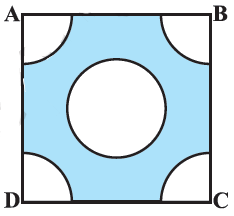# Ex.12.3 Q5 Areas Related to Circles Solution - NCERT Maths Class 10

Go back to  'Ex.12.3'

## Question

From each corner of a square of side $$\text{4 cm}$$ a quadrant of a circle of radius $$\text{1 cm}$$ is cut and also a circle of diameter $$\text{2 cm}$$ is cut as shown in the given figure. Find the area of the remaining portion of the square.Video Solution
Areas Related To Circles
Ex 12.3 | Question 5

## Text Solution

What is the known?

From each corner of a square of side $$\text{= 4 cm}$$ a quadrant of a circle of radius $$\text{1 cm}$$ is cut and also a circle of diameter $$\text{2 cm.}$$

What is the unknown?

Area of remaining portion of the square.

Reasoning:

(i) Since diameter of circle which is cut out $$\text{= 2 cm}$$

$$\therefore\;$$ Radius of this circle $$(r) =\text{ 1 cm}$$

Area of portions cut out of square $$=$$  Area of the circle $$+$$ 4$$\times$$(Area of each quadrant)

\begin{align}&= \pi {r^2} + 4\left( {\frac{{{{90}^ \circ }}}{{{{360}^ \circ }}} \times \pi {r^2}} \right)\\ &= { \pi {r^2} + 4 \times \frac{{\pi {r^2}}}{4}}\\ &= {\pi {r^2} + \pi {r^2}}\\ &= 2\pi {r^2}\end{align}

(iii) From the figure it is clear that

Area of remaining portion of the square $$=\;$$Area of square $$-$$ Area of portion cut out of square

\begin{align}= {\left( \rm{side} \right)^2} - 2\pi {r^2}\end{align}

Steps:

Diameter of circle $$\text{= 2 cm}$$

Radius of circle \begin{align} (r) = \frac{{2\,{\text{cm}}}}{2} = \,1\,{\text{cm}} \end{align}

Radius of all quadrants cut out (r)=1cm$$\rm{}(r)=1\,cm$$

Area of the portions cut out of the square $$=$$  Area of a full circle  $$+\, 4 \;\times$$  (Area of each quadrant)

\begin{align}&={\pi {r^2} + 4\left( {\frac{{{{90}^\circ }}}{{{{360}^\circ }}} \times \pi {r^2}} \right)}\\ &={ \pi {{(1)}^2} + 4 \times \frac{{\pi {{(1)}^2}}}{4}}\\ &={\pi + \pi }\\ &={2\pi }\end{align}

Area of remaining portion of the square $$=$$ Area of square $$-$$ Area of portions cut out

\begin{align}&= \rm{}{\left( {side} \right)^2} - 2\pi {r^2}\\&=\rm{} {\left( {4cm} \right)^2} - \frac{{44}}{7}c{m^2}\\&= \rm{}16c{m^2} - \frac{{44}}{7}c{m^2}\\&= \rm{}\frac{{112 - 44}}{7}c{m^2}\\&=\rm{} \frac{{68}}{7}c{m^2} \end{align}

Learn from the best math teachers and top your exams

• Live one on one classroom and doubt clearing
• Practice worksheets in and after class for conceptual clarity
• Personalized curriculum to keep up with school